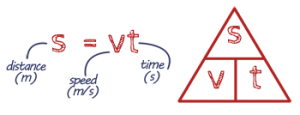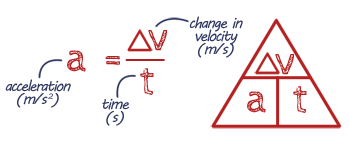# Motion

## KS3 - Motion recap

speed, motion graphs,

Websites  Videos  Games  Quizzes

BBC Bitesize - Speed distance and time

## Key Words

Distance - how far an object has moved (a scalar quantity).

Displacement - measures the distance and direction in a straight line (a vector quantity).

Vectors - have a magnitude and direction.

Scalar - only have magnitude and no direction.

Speed/Velocity - how fast an object is going (speed is a scalar, velocity is a vector).

Typical speeds for everyday objects:

 walking - 1.5 m/s car - 25 m/s running - 3 m/s train - 55 m/s cycling - 6 m/s plane - 250 m/s

Acceleration - how quickly you're speeding up.

Uniform Acceleration - constant acceleration.

Distance-Time Graph - if an object moves in a straight line, its distance travelled can be plotted on a distance-time graph.

Velocity-Time Graph - when a object's velocity changes it can be plotted in on a velocity-time graph.

Friction - slow things down. It always acts in the opposite direction to the movement.

Drag - Increases as speed increases.

Terminal Velocity - Maximum speed an object will fall at. Maximum speed depends on the shape and area of an object.

Also see Forces, Force & Motion

## Revision NotesPrint out revision notes

Need more paper?
Print more

## EQUATION Distance using speed & time## EQUATION Acceleration